## research papersJOURNAL OFSYNCHROTRONRADIATION
ISSN: 1600-5775
Volume 12| Part 2| March 2005| Pages 224-233

## Specimen charging in X-ray absorption spectroscopy: correction of total electron yield data from stabilized zirconia in the energy range 250–915 eV

aDepartment of Physics and Astronomy, University of Glasgow, Glasgow, UK, and bDepartment of Chemistry, University of Glasgow, Glasgow, UK
*Correspondence e-mail: a.craven@physics.gla.ac.uk

(Received 13 January 2004; accepted 18 November 2004)

The effects of specimen charging on X-ray absorption spectroscopy using total electron yield have been investigated using powder samples of zirconia stabilized by a range of oxides. The stabilized zirconia powder was mixed with graphite to minimize the charging but significant modifications of the intensities of features in the X-ray absorption near-edge fine structure (XANES) still occurred. The time dependence of the charging was measured experimentally using a time scan, and an algorithm was developed to use this measured time dependence to correct the effects of the charging. The algorithm assumes that the system approaches the equilibrium state by an exponential decay. The corrected XANES show improved agreement with the electron energy-loss near-edge fine structure obtained from the same samples.

### 1. Introduction

X-ray absorption spectroscopy (XAS) and electron energy-loss spectroscopy (EELS) are two closely related techniques that can be used to obtain structural and chemical information about a material. In each case the incident radiation can eject a bound atomic electron, giving rise to an absorption edge in the case of XAS or an ionization edge in the case of EELS. The fine structure in the energy region up to about 50 eV above the edge onset provides important information about the electronic, structural and chemical properties of the matter. In XAS, this is referred to as X-ray absorption near-edge structure (XANES) (Stöhr, 1992; de Groot, 1994). In EELS, the same phenomenon is described as energy-loss near-edge structure (ELNES) (Egerton, 1986; Disko, 1992). In principle, the same electronic transitions are probed by both techniques and, to a first approximation, the intensity in both is controlled by the dipole matrix element between the initial and final states. Thus the information obtained is localized to the site of the excitation by the compact nature of the initial state and provides information on the unoccupied density of states on that site with symmetries allowed by the dipole selection rule Δl = ±1. This means that an electron occupying an s orbital in the initial state will be excited into an un­occupied p-like state, while one in a p orbital will be excited into empty s- or d-like states. Hence, XANES and ELNES are probes of the site and symmetry-projected density of un­occupied electronic states around the excited atom.

In general, EELS is restricted to energy losses of less than ∼2 keV owing to the rapid decrease of the signal with energy loss. The advantage of the technique is that it can be carried out with sub-nanometre spatial resolution when the spectrometer is coupled to a modern nanoanalytical electron microscope. In contrast, XAS can be used to record the ionization edge from almost any bound electron using the high-intensity X-ray radiation available from a synchrotron facility. However, the limited focusing possible when using X-rays on a typical synchrotron beamline normally means that lateral spatial resolution in XAS is of the order of millimetres.

The simplest and most widely used detection technique in XAS experiments in the soft X-ray region is total electron yield (TEY). The TEY signal consists of all the electrons escaping from the specimen as a result of the cascade process started by the initial absorption of each photon. Close to the threshold of an edge, the contribution of that edge to the TEY signal is dominated by the contribution from the cascade process initiated by the ejected Auger electron as the photoelectron has little energy (Henke et al., 1979). However, the underlying background also has contributions arising from high-energy photoelectrons ejected from much less tightly bound atomic states, the Auger electrons from which can have much lower energy. Although other detection techniques can be used, the TEY method is popular because of the large signal available and the ease of measuring the signal via the drain current from the sample. It has been shown that TEY can be used to derive absorption coefficients (Elam et al., 1988; Henke et al., 1981; Owens et al., 1997; Erbil et al., 1988). The main disadvantages of TEY are the range of mechanisms giving rise to escaping electrons and the relatively small depth probed in the sample.

For insulating specimens, there is also the problem of sample charging. There are many papers dealing with how sample charging affects a range of techniques (e.g. Cros, 1992; Cazeau, 1999; Seah & Spencer, 2000). Charging affects the energies of electrons and hence causes major shifts of features in X-ray photoemission spectroscopy (XPS) and Auger electron spectroscopy (AES). However, in studying XANES, the energy is defined by the incident radiation and so the effect is on the intensity of the signal collected when using TEY (Gilbert et al., 2000). In the cases of ELNES, the energy lost by the primary energy is measured. Provided that the same current is incident on the specimen while the spectrum is recorded, the charge on the specimen is constant. This charge will cause the primary electron to lose a little kinetic energy before reaching the specimen but it will regain the same amount leaving the specimen. Thus neither the energy nor the intensity of a feature in ELNES is affected by equilibrium charging.

A widespread method of avoiding any charging effect at insulating materials is the performance of XAS measurements by TEY detection in the presence of an inert gas atmosphere such as helium. The gas ionization process induced by the Auger electrons emitted in the decay of the X-ray-induced holes produces mobile gas-phase charges that instantly neutralize any charge accumulating on the sample. This simple technique works very well over the whole range of X-ray energies (Schroeder et al., 1998) including the soft absorption edges (Adamczyk et al., 2000). Unfortunately the configuration of the beamline available in the current study precluded the use of this technique.

If the specimen is in powder form, the effect of charging on the XANES can be reduced by mixing the insulator with graphite (Nordberg et al., 1970) or carbon black (Obrovac et al., 1997), establishing an electrically conducting network through the sample. This technique was employed here. However, in some cases this does not solve the problem, as illustrated in Fig. 1. This figure shows the as-recorded Ce M4,5-edge from a pellet composed of 35 wt% graphite and 65 wt% of 21 mol% CeO2–ZrO2. The background has been removed by subtracting the extrapolation of the signal prior to the edge. In the energy region between the M5 and M4 white lines, the signal intensity drops below the background level, i.e. the contribution from the edge apparently becomes negative. Clearly, this is an unphysical result and is a consequence of charging in the insulating grains of 21 mol% CeO2–ZrO2 despite the presence of conducting graphite powder.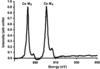Figure 1 As-recorded Ce M4,5-edge XANES from 40 wt% graphite and 60 wt% of 21 mol% CeO2–ZrO2 after background subtraction showing the non-physical negative region between the white lines resulting from specimen charging.

The graphite introduces a second problem in that it gives rise to a strong carbon K-edge in the spectrum. As discussed in §5, the XANES and the extended X-ray absorption fine structure (EXAFS) from this edge both obscure subsequent weak edges and make background subtraction more difficult for the O K-edge.

In this paper we address the correction of these effects in data obtained during a study of the XANES on the absorption edges in ZrO2 stabilized with a range of metal oxides (Y2O3, CeO2, HfO2 and La2O3). All of the XANES measurements reported here were carried out using the TEY signal. Where possible, the XANES obtained was compared with the ELNES collected from the same specimens. In general, the relative energies of the peaks observed in the two techniques are in excellent agreement. However, the relative intensities of the features differ significantly. The discrepancy is greatest in regions where the TEY signal intensity changes most rapidly during the acquisition of the XANES data, i.e. immediately after the edge onset. This is the result of the time constants of the charging process, which are large in comparison with the dwell time at each energy. By measuring the TEY signal at equilibrium at an energy immediately below an ionization edge of interest and then suddenly stepping the energy onto the edge, the time dependence of the signal from the edge can be measured. This experimentally measured time dependence can then be fitted to an analytic function which is used to correct the XANES data.

### 2. Experimental

#### 2.1. Materials

We have investigated a range of ZrO2 samples doped with 3, 5, 8, 10, 15, 20, 25 and 30 mol% Y2O3, 10 mol% La2O3, 10, 21, 50 mol% CeO2 and 10 mol% HfO2. Most of the samples were synthesized and supplied by MEL Chemicals. The 21 and 50 mol% CeO2 doped materials were supplied by Johnson Matthey. In addition, we investigated the corresponding pure oxides, i.e. ZrO2, Y2O3, La2O3, CeO2 and HfO2 (Alpha Aesar). All the samples were supplied in powder form. With the exception of the 3 and 5 mol% Y2O3–ZrO2 materials, X-ray diffraction showed them to be single phase with lattice parameters consistent with their compositions. The 3 and 5 mol% Y2O3–ZrO2 powders were intended to be the stabilized tetragonal phase. Owing to the nature of the preparation process, small volume fractions of the cubic or monoclinic phase are often found in powders with these compositions. Here the 5 mol% powder contained trace quantities of the stabilized cubic phase while the 3 mol% sample contained 5–10% of monoclinic zirconia. At this level, the presence of the second phase has no effect on the results presented here.

For the XAS experiments, each sample was mixed and ground with graphite powder (Carbone of America, Ultra Carbon Division), then pressed into a disc using a hydraulic press, giving discs that were 1 mm thick and 13 mm in diameter. The discs of Y2O3–ZrO2, CeO2–ZrO2, HfO2–ZrO2 and La2O3–ZrO2 were formed using 40 wt% graphite. In order to ensure that the volume fraction was approximately constant, the amount of graphite mixed with the pure oxides varied: 35 wt% (CeO2), 25 wt% (HfO2), 40 wt% (La2O3) and 43 wt% (Y2O3). For the EELS experiments, the samples were ground in a mortar, dispersed in propanol and then agitated in an ultrasonic bath. Droplets of the suspension were then placed on a holey carbon-film-coated copper grid.

#### 2.2. XAS procedure

The XAS experiments reported here were carried out at the Daresbury synchrotron radiation source using beamline 1.1. Beamline 1.1 is a soft X-ray beamline providing monochromatic photons over the energy range 200–1000 eV. In the experiments, a spherical-grating monochromator with 1800 lines mm−1 was used. Two-thirds of the grating was coated in gold and one-third in nickel. The nickel-coated section is used to reduce the second-order light content at energies above ∼425 eV, i.e. half of the Ni L3-edge threshold (∼850 eV). For the oxygen K-edge experiments, data were recorded over the energy range 250–630 eV using the Ni-coated region of the monochromator. The energy range was chosen so that both the carbon and oxygen K-edges were present in the spectra obtained. The carbon K-edge is required for a number of reasons, as discussed below. On beamline 1.1 we also performed measurements at the La and Ce M4,5-edges over the ranges 790–950 eV and 840–1000 eV, respectively. For these edges, the Au-coated area of the grating was used.

The TEY signal (Ie) was detected using an electrometer connected to the isolated sample holder, which was biased at −56 V with respect to ground. In the presence of charging, this bias voltage plays an important role in keeping the signal level high. The incident-beam intensity (Io) was monitored by measuring the drain current from a fine gold mesh biased at −47 V. This mesh was thoroughly ion-beam cleaned to remove surface contamination. There was no window (contamination barrier) between the gold mesh and the sample. The voltage outputs from the electrometers were converted to frequencies using voltage-to-frequency converters and the output pulse trains fed to counters that were enabled for the integration time chosen. All spectra were recorded with an integration time of 1 s per point. To minimize the time required to scan a spectrum, it was divided up into regions whose step size was matched to the detail present in the signal. The set-up used for the carbon and oxygen K-edges is shown in Table 1.

 Table 1Energy step used in each energy range when recording the C and O K-edge XANES
Einitial (eV) Step (eV) Efinal (eV)
250.00 1.00 280
280.20 0.20 350
352.00 2.00 430
430.50 0.50 500
500.10 0.10 570
570.25 0.25 590
590.50 0.50 620

If the spectra obtained are to be corrected for the effect of specimen charging, it is important to be aware of the `real' acquisition time per point. While the signal integration time was 1 s, the average dwell time per point, i.e. the total acquisition time divided by the total number of points, was 2 s. The difference between the integration and dwell times is due to the time required for the monochromator to settle at each new angular position. In addition, the charge state of the sample should ideally reach equilibrium before the start of the acquisition sequence to aid charge correction. To approach this, the monochromator was positioned at the starting energy and the specimen was irradiated at this energy for a period of at least 3 min before a scan was commenced.

Preliminary investigations also revealed that the XANES spectrum from an as-pressed pure graphite disc exhibited an oxygen K-edge of significant intensity. If the surface of the graphite disc was gently abraded by rubbing on filter paper, the oxygen signal was reduced to a low level. The spurious oxygen signal is clearly associated with the surface of graphite since it disappears when the surface layer is removed. However, there was no evidence of a rapid build-up of oxygen on the surface again if rubbed specimens spent time in air prior to insertion into the vacuum of the beamline. This suggests that the surface oxygen results from the disc-pressing process and is presumably there in all the specimen discs. To remove it, all discs were rubbed in this fashion prior to recording data. Fig. 2compares the O K-edge from a rubbed and an unrubbed disc made up from 70 wt% graphite and 30 wt% of 10 mol% Y2O3–ZrO2, showing that removal of this surface layer is crucial. In order that the residual contribution from the oxygen in the graphite can be neglected, it is important to have a high volume fraction of YSZ in the pellet. A pellet with 70 wt% graphite contains only ∼14 vol% of YSZ. Thus all subsequent work was performed with pellets containing 40 wt% graphite, which is equivalent to 37 vol% YSZ. Going to lower graphite fractions appeared to lead to a significant increase in the problems from charging.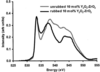Figure 2 Comparison of the XANES O K-edge from a sample made up of 70 wt% graphite and 30 wt% of 10 mol% Y2O3–ZrO2, before and after removing the surface layer by rubbing on filter paper. The spectra are normalized to match at the first peak.

#### 2.3. Measurement of specimen charging in XAS

To monitor the extent of the charging effect and enable the time constants for the process to be determined, the response of the TEY signal to a sudden change in its magnitude is required. More specifically, the response of the signal from the material of interest must be identified. Thus the time response of an ionization edge is measured. This is achieved by measuring the TEY signal, Ie, as a function of time using the time scan mode of acquisition. In this mode the incident energy is kept constant and the signal is repeatedly measured at fixed integration time. This time scan mode was modified so that, after a predetermined number of measurements at one incident energy, the energy was changed and a further sequence of intensities measured immediately after the change. By choosing an energy in the background region immediately before an absorption edge from the material of interest and one at the highest point on the edge, a large change in Ie is obtained. This time variation is dominated by that of the ionization edge since the change in the background contribution is very small over this energy step. For example, in the case of the oxygen K-edge, an initial energy of ∼531 eV might be chosen, i.e. just prior to the onset of the absorption edge. Ie is measured for 50 integrations, each of 3 s. The photon energy is then changed to a value of ∼533 eV (i.e. on the maximum of the O K-edge) in the shortest time the system will allow (∼1 s). Ie is then measured for a further 50 integrations, each of 3 s. Prior to starting the scan, the system is allowed to settle at the initial energy for a time longer than the time spent acquiring data at each energy. Fig. 3shows one such time scan recorded from the 3 mol% Y2O3–ZrO2 sample. This is similar to the shape observed by Gilbert et al. (2000). At this point it should be noted that supplementary time scan measurements, using both the zirconia samples and a pure graphite sample, showed that there is no time dependence from the change of the signal from the graphite.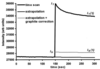Figure 3 The time response of the total electron yield signal, Ie, initially close to equilibrium at 531 eV (the background prior to the O K-edge) when the energy is changed to suddenly changed 533 eV (the O K-edge maximum) after 150 s (I1). Also shown are the extrapolated background signal and the extrapolated background contribution corrected for the change in the graphite signal (Ib), as discussed in §5. The sample is made up of 40 wt% of graphite and 60 wt% of 3 mol% Y2O3–ZrO2.

The accuracy of any correction procedure can only be validated by an independent check. As discussed above, the intensities in ELNES are not affected by any steady-state charging of the specimen. Hence the corrected XANES is compared with the ELNES from the same material.

#### 2.4. EELS procedure

All the EELS measurements were carried out using a 100 kV HB5 scanning transmission electron microscope (STEM) fitted with post-specimen lenses (Craven & Buggy, 1981) and equipped with a GATAN 666 PEELS spectrometer and a Link Analytical energy-dispersive X-ray (EDX) spectrometer. The probe half-angle was 11 mrad, giving a probe diameter of ∼1 nm and a probe current of ∼0.2 nA. The collection semi-angle at the specimen was 12.5 mrad. During spectrum acquisition, the probe was scanned over an area 5.2 nm × 3.8 nm to reduce the electron dose to the specimen. Details are given by Vlachos et al. (2001).

EDX spectroscopy was used to determine the dopant distribution in each sample. Preliminary results revealed that, while the composition within individual grains in a sample appeared to be homogeneous, there was significant compositional inhomogeneity between different grains in some of the powders (Vlachos et al., 2001). For this reason, an EDX spectrum was recorded from the same area as each set of EELS data to determine the local dopant concentration.

In the case of the CeO2–ZrO2 materials, significant radiation damage was observed. In this case, a sequence of spectra was acquired from an area that had only been imaged at relatively low magnification. Hence it had received an initial dose far lower than that required to record a spectrum. The beam blank system was used to stop the incident electrons reaching the specimen while the magnification was increased and the beam shift settings changed to previously determined values that placed the area of interest at the correct position. After the spectrometer had completed a detector readout, the beam was unblanked and the sequence of spectra recorded. By comparing the shapes of successive spectra in such a sequence, it was possible to identify the point at which a shape change was starting to occur and hence to make use of only those spectra acquired before that point was reached.

### 3. Modelling the charging process

As described above, time scan measurements were performed to investigate the charging process. The purpose of these measurements was to measure the time response of the TEY signal, Ie, at fixed energies, E1 and E2, prior to and on the absorption edge, respectively. The time scan in Fig. 3shows that Ie varies slowly with time at the initial energy of 531 eV. Thus, even after a settling time of 3 min prior to starting the scan, the initial signal is still changing with time. Part of this is the steady decrease of the current in the synchrotron itself and part is the sample still coming to equilibrium as a result of charging processes with very long time constants. The direction of this residual settling depends on whether Ie at 531 eV is greater or less than at the previous energy of irradiation. The rate of change depends on the time the sample has been at 531 eV before the time scan was started and also on the sample, since the time constants vary from sample to sample. However, a long time constant has no effect on sharp features recorded over times that are short in comparison, and so these slow variations are neglected.

In order to correct the XANES measurements for charging, it is necessary to have a quantitative description of the time-dependence of the TEY signal. To obtain a relatively simple correction procedure for a signal that is the result of a complex cascade process, a number of simplifying assumptions are made. Consideration of their limitations is discussed when the results are presented in later sections. As a first approximation, the sample is modelled as a capacitor. One electrode is connected to ground via an electrometer and a source of bias voltage, Vb, and the other is in a vacuum and exposed to the incident photons. The latter electrode is taken to be sufficiently thin that it stops neither photons nor electrons. It is assumed that the dielectric gives a large but finite resistance between the plates. While highly idealized, this reproduces the situation in the powder sample reasonably well. At some points on a particle, the graphite is in contact with the surface while at others there is no direct contact with the graphite. However, any such isolated point on the surface of the particle will have a resistance (either surface or bulk) to the graphite. This resistance will allow any surface charge to dissipate eventually. Cazeau (1999) and Gilbert et al. (2000) have used the same basic approach to this problem.

The effect of the photon beam is to eject electrons from the exposed surface into the vacuum and equilibrium will occur when the ejected electrons are replaced by electrons flowing in from the graphite at the same rate. To create this current flow, the potential of the surface must increase relative to the graphite. This, in turn, will mean that only electrons with kinetic energy in excess of the potential barrier created will escape into the vacuum and so the number escaping will decrease as the surface charge increases. At some surface potential distribution, equilibrium is reached.

The inset in Fig. 4shows the equivalent circuit where a photon flux causes ejection of electrons from the surface at a rate corresponding to a conventional current, Ie, flowing onto the top plate of the capacitor. This results in a current, Ie, flowing through the electrometer to ground. Ie is made up of two components. Ic increases the charge, q, on the capacitor while IR flows through the resistance between the plates. A bias voltage, Vb, is applied to the lower plate. The voltage of the top surface with respect to ground is Vs. As the capacitor gains charge, q, the potential difference across the plates, Vc = (VsVb), rises and the current through the resistance, R, between the plates increases. At equilibrium, the voltage of the top surface is Vso, Ic is zero and IR is equal to Ie.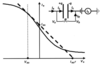Figure 4 A schematic diagram showing the variation of Ie with the surface potential Vs. The equivalent circuit for the charging process is shown in the inset.

Fig. 4shows schematically the variation of Ie with Vs at constant incident photon energy and intensity. The secondary electrons emitted from the surface will give the curve a shape similar to that from a thermionic diode or vacuum photodiode, i.e. Ie rises to saturation as Vs becomes negative and falls as Vs becomes positive. To achieve cut-off, Vs would have to rise to a value consistent with the energy of the most energetic electron created in the absorption process.

nothi

One approach to finding a simple functional form for the process is to assume that the curve in Fig. 4can be approximated as a straight line over the range of parameters encountered. Thus Ie = Ieo-Vs/r where r is a dynamic resistance, given by the inverse of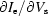evaluated at the equilibrium value of Vso.

The magnitude of Ie depends on the incident flux and also on the incident photon energy via the absorption coefficient in the material. If either the incident flux or the absorption coefficient increases, the curve increases in height and possibly changes in shape. If the material is insulating so that R is large, an increase in flux or absorption will result in an increase in the charge on the capacitor. This increases Vc and hence Vs, resulting in a drop in Ie until equilibrium is re-established at a new value of Vso.

If it is assumed that r remains constant, it is possible to show that a sudden change in photon energy across an absorption edge, as in Fig. 3, will result in an initial step increase in the TEY signal followed by an exponential decay to the equilibrium value so that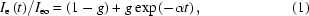where Ieo is the initial change of the drain current to ground and Ie(t) is the change a time, t, later. α is an inverse time constant and g is a measure of the magnitude of the charging.

However, r will vary with Vs. Moreover, R and the capacitance will vary from particle to particle depending on the size and the contact made by the surrounding graphite. Cazeau (1999) notes this problem and Gilbert et al. (2000) develop an implicit expression for the current density as a function of time for an idealized system. However, their expression cannot be used as a simple fitting function to describe the experimental time dependence. It is clear that equation (1)is unlikely to describe the experimental observations sufficiently well. However, since only an accurate parameterization of the time variation is required below, equation (1)was simply extended to allow for more than one time constant giving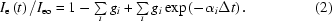Using two time constants, equation (2)resulted in an excellent agreement with the experimental data, as shown in Fig. 5. Here the experimental points are derived from the data in Fig. 3by forming the reduced quantity [I1(t)-Ib(t)]/(I1-Ib) where Ib(t) is the extrapolated background signal corrected for any change in the graphite signal, as discussed in §5. The deviation between the experimental data and the fit is less than 0.4%, as shown in the inset.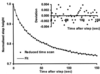Figure 5 Fit of equation (2)with two sets of charging constants to the reduced form of the data in Fig. 3obtained by forming the quantity [I1(t)-Ib(t)]/(I1-Ib) where Ib(t) is the extrapolated background signal after correction for the graphite signal. The deviation between the experimental data and the fit is shown in the inset.

Typical values for the two time constants of the O K-edge are 15 s and 100 s but the values obtained for a given sample can vary by up to 25% depending on the area of the sample irradiated. There is also some dependence of the time constants on the Y2O3 fraction, with the end members of the series (0% and 30% Y2O3) having the shortest time constants while the mid-range compositions have the longest. Thus the charging behaviour is clearly a local property of the particular specimen as well as a property of the material itself.

### 4. An algorithm for correcting for the effect of charging

Having established a simple expression for the time dependence of the charging process, a scheme to correct for the effects of charging can be developed. In the absence of charging, the signal is independent of time unless the energy of the incident radiation changes. Thus if the scan process is approximated to a series of steps in energy, the signal observed would be of the form of Iu(t), as shown schematically in Fig. 6for the case where the signal increases with energy. If the specimen charges, then the signal will reach the level appropriate to the equilibrium charge state of the specimen if the energy is kept constant for a period long compared with the longest time constant of the charging process. This equilibrium signal would have the form of I(t) in Fig. 6. The time constants of 15 s and 100 s are long compared with the time spent at each energy in the scan (∼2 s). Indeed to allow the signal to reach equilibrium at each point would require an increase in the time per scan from ∼1 h to >>50 h.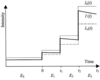Figure 6 Schematic diagram showing the signal obtained as the energy is incremented for three conditions: in the absence of charging, Iu(t) (fine line); with a charging sample coming to equilibrium at each energy step, I∞(t) (dashed line); when the sample charges over a period that is long compared with the time at each energy, I(t) (thick line).

Equation (2)shows that the charging process will result in I(t) approaching the equilibrium value, I(t), in a way that can be described by a sum of exponentially decaying terms. However, for the purpose of deriving the form of the correction necessary, it is assumed that the effect of the charging process can be represented by a single exponential term. The additional time constants will be included at the end as a simple extension to the procedure.

If the system starts in equilibrium at energy E0, and the energy suddenly changes to E1, then there will be an initial change in the signal,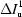. This will be the same change that would have occurred in the absence of charging since the sample has not had time to change its charge state. As the sample charges, the signal will change with time according to (1)giving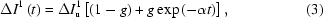which will eventually decay to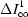=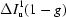. Thus, at time t, the signal is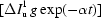from the equilibrium value. If after a time interval Δt the energy is changed from E1 to E2, the signal will increase by a further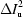. Again this is the change that would have occurred in the absence of charging. However, the new equilibrium value will be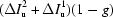above the value at E0. Hence the signal will differ from the new equilibrium value by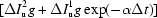. The difference from the new equilibrium value will continue to decay exponentially so that, at time t after the energy first changed from E0 to E1, it will be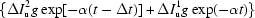. Each additional energy step will add a further deviation from equilibrium. Thus the overall deviation of the signal from the current equilibrium value will be a sum of terms. Each term will be the product of the initial signal change caused by the energy step,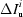, multiplied by an amplitude term, g, and further multiplied by an exponential factor whose exponent is determined by the time elapsed since the energy step occurred. Hence for a series of energy steps at time intervals of Δt,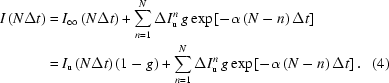For the charging process, αΔt is much less than unity and so the sum can be replaced by an integral, allowing easier manipulation. For this, nΔt is replaced by t′, the time at which the energy change occurred, and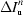is replaced by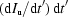where the derivative is evaluated at t′ giving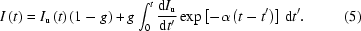This can be integrated by parts and re-arranged to give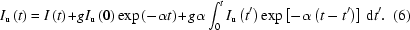Thus the signal, Iu(t), that would have been obtained in the absence of charging can be obtained from the measured signal, I(t), by the addition of two correction terms since the parameters g and α, which describe the effects of the charging, can be found from the time scan.

The first correction term can be evaluated directly at time t, since Iu(0) is just the equilibrium signal before the start of the scan,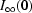, divided by (1 − g). However, the second term involves the values of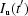up to the current time, t. By starting with Iu(0), the value of Iu(t1) can be evaluated. Using this, the value of Iu(t2) can be evaluated and so on. Since the data obtained are discrete, equation (6)is replaced by a sum so that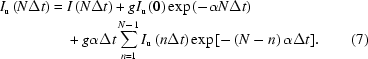Here the upper limit of the sum is (N − 1) since it only involves values prior to the current time.

If the time response of the signal involves more than one time constant, equation (3)can be generalized to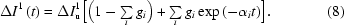The linear form of the equations then allows (7)to be re-written as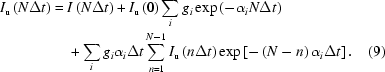In principle, the algorithm should be applied to data that have an equilibrium value of the signal in the first channel to give the true corrected signal. However, if the algorithm is applied starting at an arbitrary point in the scan, the corrected signal approaches the true corrected signal after a number of data points which corresponds to an acquisition time of several times the longest time constant involved.

### 5. Dealing with the background

In the experimental data, Ie/Io, the edge of interest sits on a background due to excitations of less tightly bound electrons in both the material of interest and the graphite. If the edge of interest is sufficiently far from other edges, the background preceding it is smooth and relatively slowly varying. By fitting a power-law function in the region before the edge, the function can be extrapolated under the edge and subtracted.

Provided there is no element on the surface of the optics having an edge in this vicinity, the change of Io with photon energy, and hence with time, will be slow. Thus the part of Ie giving rise to the background will also change slowly with time. Hence, charging will have little or no effect on the background as equation (5)shows that the effect of charging is controlled by dIe/dE. Thus, under these circumstances the background can be removed either before or after correcting for the effects of charging. These conditions are satisfied for all edges of interest here apart from the O K-edge.

For the O K-edge, neither condition is satisfied since the EXAFS from the C K-edge perturbs the background shape while the oxygen on the beam optics causes a significant variation of Io in the vicinity of the edge. The effect of the EXAFS can be removed by recording the C K-edge from a pure graphite specimen, scaling it to match the C K-edge from the zirconia-containing specimen and subtracting. In principle, this gives the O K-edge signal from the zirconia alone. The effects of charging must be corrected at this point and the background from the zirconia removed subsequently. The following procedure was used for the background subtraction. The spectrum of the pure carbon sample was scaled and subtracted from that of the zirconia sample. The shape of the Zr and Y M2,3-edges near to 330 eV and 300 eV, respectively, allow the correct scaling factor to be determined. It is then possible to fit a reasonable power-law background in the region prior to the O K-edge and extrapolate it under the edge to remove the background. The result still depends on the fitting window used but to a far lesser extent than if the graphite contribution had not been removed.

Returning to the time scan of Fig. 3, the initial change in Ie caused by the change in energy is composed of two parts, one from the graphite and one from the zirconia. The part from the graphite has no time dependence and, to find the change owing to the contribution from the zirconia alone, a correction must be made to the background signal. The values of Ie/Io for the graphite at the two energies in the time scan are known from the scaled graphite spectrum discussed in the previous paragraph and these values are very similar. However, the value of Ie itself changes significantly because of the change of Io resulting from oxygen on the optics. Thus the change in Ie from the graphite can be obtained simply by multiplying the value of Ie/Io from the graphite by the change of Io over the energy step in the time scan. This change in Ie from the graphite is then used to correct the base line of the time scan, as shown in Fig. 3.

### 6. Correction of the effects of charging

#### 6.1. Ce and La M4,5-edges

In the case of La and Ce M4,5-edges, the time scans were performed on the M5-edges. The time scans were fitted using two time constants. Spectra from a pure graphite sample showed that the background was indeed smooth across the edges. The data were charge corrected and the background removed subsequently. Fig. 7compares the Ce M4,5-edges from the 21 mol% CeO2–ZrO2 sample recorded by XAS before and after charge correction. (The uncorrected data were shown in Fig. 1.) The spectra are normalized in the region from 80 to 110 eV after the M5 white line. In the corrected spectrum, the white-line intensity is reduced and the unphysical negative region between the white lines is removed.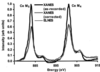Figure 7 The Ce M4,5-edge XANES from 40 wt% graphite and 60 wt% of 21 mol% CeO2–ZrO2 before and after correction for specimen charging compared with the ELNES from the Ce M4,5-edge from 21 mol% CeO2–ZrO2. All have had the background subtracted.

Also shown are the edges recorded by EELS normalized to the Ce M5 peak intensity. It is quite clear that the agreement between the charge-corrected XANES data and the ELNES data is excellent, showing that the charge-correction algorithm works well for this edge. There are two areas of minor discrepancy. The first is that the continuum in the EELS data is high in the range 910–940 eV. This may be related to residual multiple scattering left by the deconvolution procedure used in processing the EELS data. The procedure assumes that the data are taken from an area of uniform thickness. In practice, the electron beam is scanned over a small area of the specimen during acquisition. Since the doped zirconia is in the form of small particles, this means that signals from a range of thicknesses may contribute to the spectrum and could perturb the deconvolution slightly. There is a second minor discrepancy. The intensity of the high-energy shoulders of the white lines in the fully corrected XANES is higher than in the ELNES while the reverse is true for the low-energy shoulders. This material undergoes radiation damage in the electron beam and one effect of this is to reduce the intensity of the high-energy shoulder and increase that of the low-energy shoulder. Thus the EELS data are probably showing the early stages of radiation damage.

#### 6.2. The O K-edge

For the O K-edges, two time constants were again used to fit the times scans. Once the graphite contribution to the spectrum has been removed, as described in §5, the algorithm for correcting the effects of charging is applied. Fig. 8compares the XANES O K-edge shape from the 25% Y2O3–ZrO2 sample after background subtraction for the following cases: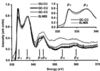Figure 8 Comparison of the O K-edge XANES from 40 wt% graphite and 60 wt% of 25 mol% Y2O3–ZrO2 at each stage of processing. GU-CU: graphite uncorrected, charged uncorrected; GC-CU: graphite corrected, charged uncorrected; GC-CC: graphite corrected, charged corrected. Also shown is the ELNES from 25 mol% Y2O3–ZrO2. All have had the background subtracted.

(a) without correction for the graphite contribution or charging (GU-CU);

(b) after correction for the graphite contribution but without correction for charging (GC-CU);

(c) after correction for both the graphite contribution and charging (GC-CC).

The O-K XANES is characterized by a number of peaks, as shown in Fig. 8. The edges have been normalized at the second peak, p2, at about 538 eV. The effect of the graphite subtraction has little effect on the shape here whereas the charge correction significantly lowers the intensity of the first peak p1 and also that of peak p3.

The ELNES shape is also plotted in Fig. 8, again normalized to p2. There is excellent agreement with the intensities of p1 and p2 in the charge-corrected XANES spectrum and the ELNES spectrum. In addition, the shapes of p3 and p4 are in better agreement than with the data uncorrected for charging. There is again a significant difference in the overall intensity between 540 and 570 eV, after which there is reasonable agreement given the uncertainties associated with background extrapolation. As well as the possibility of a residual error from the deconvolution process in the EELS data, there is a further potential source of difference in this energy region. The grid used to monitor Io is made of gold, which has an N5-edge at 545 eV. This will cause a small increase in Io with no corresponding increase in Ie and hence a reduction in Ie/Io. The effect of this reduction will be magnified by the background-subtraction process and partially explains the lower XANES signal in Fig. 8.

The agreement between the p1/p2 intensity ratio in the ELNES and that in the XANES always improved after correction for charging. In general, the XANES for a given material showed a systematic increase in this ratio the later the data were taken in the experimental run, so that the agreement with the ELNES became worse. The later the data were taken, the bigger the change. The factor which correlates most strongly with this observation is the time since the cleaning of the gold mesh used to monitor Io. There is a significant change with time of the shape of the Io versus E curve in the vicinity of the O K-edge and the effect may be the result of a small error in the normalized ratio Ie/Io intensity resulting from this. Confirmation would require a more detailed investigation of the effect.

### 7. Discussion

It is clear that there are a large number of factors that can perturb the XANES from these highly insulating materials. Fig. 2showed that surface oxygen can play a major role if not removed. While all samples were treated in the same way, there is no guarantee that all had this spurious oxygen removed to the same degree. However, the samples were not examined in order of composition but followed the general trend of 0, 5, 10, 20, 30, 3, 8, 15, 25 mol% Y2O3 to ensure that the whole range of compositions was covered in the event of a problem with the SRS limiting the time available. In addition to these compositions, the specimens containing the other dopants were also examined. At least two spectra were recorded from each sample at different times in the experimental session. The scatter at each composition is related to the systematic growth of contamination on the gold grid used to measure Io. Data taken later in the session show bigger discrepancies from the equivalent ELNES.

Given the particle-to-particle variation in the Y/(Y+Zr) ratio in the 20 and 25 mol% Y2O3–ZrO2 samples, it would be expected that p2 in the XANES from these materials, which averages over many particles, would be wider than that from the ELNES, for which only data with Y/(Y+Zr) close to the nominal values are included. There is some indication of this in the inset of Fig. 8where the minimum between p1 and p2 is shallower in the XANES than in the ELNES. This could be the result of the broadening of p2 but confirmation of this would need additional work.

While the charge-correction algorithm works well near the threshold from which the time scan was recorded, there is evidence that it works less well away from the threshold. Some small background artefacts were observed when the scan rate changed or when the second-order radiation was suddenly removed at 430 eV by the Ni coating on the grating. These artefacts occur because the processes generating the TEY signal from the background differ significantly from those generating the TEY signal from the O K-edge. At the threshold of the O K-edge, the main contribution to the TEY signal from O is from the O Auger electrons, with the photoelectrons having little energy to create secondary electrons. On the other hand, the main contribution to the TEY signal from the background prior to the O K-edge is from excitation of the Zr M4,5-edges since the contribution from the graphite has already been removed. Here the first-order radiation creates photoelectrons and Auger electrons of similar energy. When second-order radiation is present below 430 eV it will create additional photoelectrons of much higher energy than the Auger electrons. Thus it is not surprising that α and g determined specifically for the O K-edge near its threshold do not describe the behaviour of the background exactly, leading to the small artefacts observed.

Finally, Gilbert et al. (2000) have investigated the illumination of the specimen with light whose photon energy is bigger than the band gap of the material but less than the work function. In principle, this introduces photoconductivity without additional signal and so reduces the charging. They showed an effect on their highly insulating biological specimens but not sufficient to stop the charging. However, it is certainly worth following up for material like zirconia.

### 8. Conclusions

XANES from an insulating powder recorded by the TEY method can show a number of artefacts, particularly those related to charging. To obtain reasonable data it is essential to bias the sample negative with respect to ground. It is also essential to mix the insulating material with a finely divided conductor whose ionization edges will not interfere with the edges to be measured from the material of interest. However, the volume fraction of material should be high enough to maximize the signal from the edges of interest but not so high as to exacerbate the effects of charging by causing flashover-type effects on its surface. It is crucial that any surface contaminants that interfere with the edge of interest are removed, oxygen being of most concern here. It was found that the hydraulic press used to make the pellets introduces a surface layer of high oxygen content and this has to be removed.

The contribution from the graphite used to minimize the charging can be removed by scaled subtraction of a spectrum from pure graphite. The time dependence of the charging process can be determined from a time scan in which the energy is switched from just below the edge of interest to the maximum intensity on the edge at a point in the middle of the scan. This time dependence can be parameterized and used in an algorithm that corrects for the effects of the charging. This procedure is satisfactory close to the edge threshold but is only approximate elsewhere.

Even after all the corrections were made, there were still systematic differences between the ELNES and the XANES. There was also a deviation in overall intensity rather than shape in the region 10–50 eV above the edge. One contribution to this is the difficulty of correcting for the multiple scattering in the ELNES when the sample thickness is non-uniform. Other contributions may come from residual artefacts in the XANES from the change in the energy of the photoelectron as a function of the energy in the spectrum or from the effect of an absorption edge in the monitor grid or from the effect of contamination in the monitor grid. Another important difference between XANES and ELNES is that the first technique averages the intensity over a large area of the sample while the latter can be used to record spectra from individual particles owing to its inherent high spatial resolution.

As methods of calculation improve, it is essential that such artefacts in the experimental data are removed so that it is clear where the remaining differences lie. It is also important that inter-comparison of different XANES measurements, or comparisons of XANES and ELNES, from insulating materials take into account the effects of charging if the XANES are recorded using the TEY method

Overall, given the number of effects involved in the XANES method, ELNES must be considered a simpler and more reliable technique for these relatively low-energy edges provided that suitable thin specimens can be made and that radiation damage is not a limiting factor. However, where signal-to-noise ratio, energy resolution and radiation damage are the key factors, XANES offers significant benefits provided the artefacts can be corrected.

### Footnotes

Now at Department of Physics, University of Ioannina, Greece.

§Now at Department of Materials, Imperial College, London, UK.

### Acknowledgements

The authors would like to thank Ian Kirkman and Sunil Patel (Daresbury), Jim Gallagher and Sam McFadzean for their help in this work. We are grateful to EPSRC (GR/L66953), MEL Chemicals and Johnson Matthey for their support in carrying out this work

### ReferencesJOURNAL OFSYNCHROTRONRADIATION